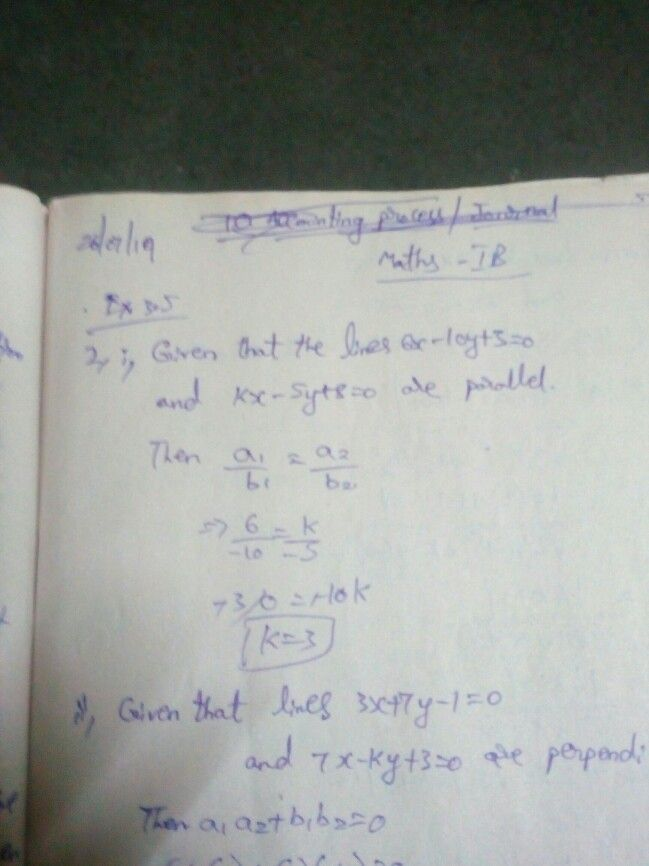Symbol
Problem$1.$ The locus of the point which is at a distance $5$ units from $\left(-3,4\right)$ $1s$ $1\right)x^{2}+y^{2}+6x-8y=0$ $2\right)$ $x^{2}+y^{2}-6x+8y=0$ $3\right)$ $x^{2}+y^{2}+6x-8y+25=0$ $4\right)$ $x^{2}+y^{2}$ $-6x+8y+50=0$
1st-6th grade
Geometry
SolutionQanda teacher - Ravitiwari